• python中的pow函数的功能是计算x的y次幂。本篇文章将带大家一起了解一下，pow()函数在Python中的用法。感兴趣的朋友了解一下。以下是 math 模块 pow() 方法的语法:import mathmath.pow( x, y )内置的 pow() 方法pow...
python中的pow函数的功能是计算x的y次幂。本篇文章将带大家一起了解一下，pow()函数在Python中的用法。感兴趣的朋友了解一下。以下是 math 模块 pow() 方法的语法:import mathmath.pow( x, y )内置的 pow() 方法pow(x, y[, z])函数是计算x的y次方，如果z在存在，则再对结果进行取模，其结果等效于pow(x,y) %z注意：pow() 通过内置的方法直接调用，内置方法会把参数作为整型，而 math 模块则会把参数转换为 float。参数：x -- 数值表达式。y -- 数值表达式。z -- 数值表达式。返回值：返回 xy(x的y次方) 的值。以下展示了使用 pow() 方法的实例：一、在命令行中的使用1、pow(x,y):这个是表示x的y次幂。>>> pow(2,4)16>>>2、pow(x,y,z)：这个是表示x的y次幂后除以z的余数>>> pow(2,4,5)1>>>二、在IDE中的使用#!/usr/bin/python# -*- coding: UTF-8 -*-import math   # 导入 math 模块print "math.pow(100, 2) : ", math.pow(100, 2)# 使用内置，查看输出结果区别print "pow(100, 2) : ", pow(100, 2)print "math.pow(100, -2) : ", math.pow(100, -2)print "math.pow(2, 4) : ", math.pow(2, 4)print "math.pow(3, 0) : ", math.pow(3, 0)
展开全文• python中的pow函数的功能是计算x的y次幂。本篇文章将带大家一起了解一下，pow()函数在Python中的用法。感兴趣的朋友了解一下。以下是 math 模块 pow() 方法的语法:import mathmath.pow( x, y )内置的 pow() 方法pow...
python中的pow函数的功能是计算x的y次幂。本篇文章将带大家一起了解一下，pow()函数在Python中的用法。感兴趣的朋友了解一下。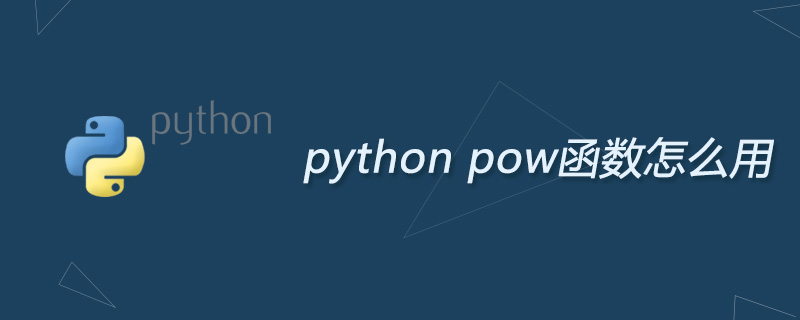以下是 math 模块 pow() 方法的语法:import mathmath.pow( x, y )内置的 pow() 方法pow(x, y[, z])函数是计算x的y次方，如果z在存在，则再对结果进行取模，其结果等效于pow(x,y) %z注意：pow() 通过内置的方法直接调用，内置方法会把参数作为整型，而 math 模块则会把参数转换为 float。参数：x -- 数值表达式。y -- 数值表达式。z -- 数值表达式。返回值：返回 xy(x的y次方) 的值。以下展示了使用 pow() 方法的实例：一、在命令行中的使用1、pow(x,y):这个是表示x的y次幂。>>> pow(2,4)16>>>2、pow(x,y,z)：这个是表示x的y次幂后除以z的余数>>> pow(2,4,5)1>>>二、在IDE中的使用#!/usr/bin/python# -*- coding: UTF-8 -*-import math   # 导入 math 模块print "math.pow(100, 2) : ", math.pow(100, 2)# 使用内置，查看输出结果区别print "pow(100, 2) : ", pow(100, 2)print "math.pow(100, -2) : ", math.pow(100, -2)print "math.pow(2, 4) : ", math.pow(2, 4)print "math.pow(3, 0) : ", math.pow(3, 0)
展开全文• Python pow() 函数描述pow() 方法返回 xy(x的y次方) 的值。语法以下是 pow() 方法的语法:import mathmath.pow( x )注意：pow()是不能直接访问的，需要导入 math 模块，通过静态对象调用该方法。参数x -- 数值表达式...
Python pow() 函数描述pow() 方法返回 xy(x的y次方) 的值。语法以下是 pow() 方法的语法:import mathmath.pow( x )注意：pow()是不能直接访问的，需要导入 math 模块，通过静态对象调用该方法。参数x -- 数值表达式。y -- 数值表达式。返回值 返回 xy(x的y次方) 的值。实例以下展示了使用 pow() 方法的实例：#!/usr/bin/pythonimport math   # This will import math moduleprint "math.pow(100, 2) : ", math.pow(100, 2)print "math.pow(100, -2) : ", math.pow(100, -2)print "math.pow(2, 4) : ", math.pow(2, 4)print "math.pow(3, 0) : ", math.pow(3, 0)以上实例运行后输出结果为：math.pow(100, 2) :  10000.0math.pow(100, -2) :  0.0001math.pow(2, 4) :  16.0math.pow(3, 0) :  1.0
展开全文• Python中，两个点之间的距离函数可以表示为math.sqrt(dx ** 2 + dy ** 2)。但是，这个函数中的每一项都有不同的表达方法：dx ** 2、 math.pow(dx, 2)和 dx * dx。有趣的是，它们的运行结果各不相同，我想知道它们...
最近，我在写一个算法来解决一个编码难题，这个难题涉及到在一个笛卡尔平面上找到一个与其他所有点的距离最小的点。在Python中，两个点之间的距离函数可以表示为math.sqrt(dx ** 2 + dy ** 2)。但是，这个函数中的每一项都有不同的表达方法：dx ** 2、 math.pow(dx, 2)和 dx * dx。有趣的是，它们的运行结果各不相同，我想知道它们是如何以及为什么会是这样的。计时测试Python提供了一个名为 timeit 的模块来测试性能，这使得测试这些表达式的运行时间相当简单。将 x 设置为 2，我们就可以对上面的三个选项进行计时测试: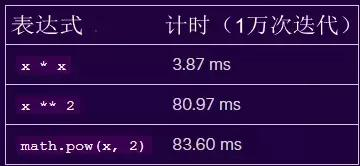表达式反汇编Python还提供了一个名为 dis 的模型，它可以对代码进行反汇编，这样我们就可以看到每一个表达式在底层做些什么，这有助于我们理解其性能差异。乘法使用 dis.dis(lambda x: x * x)，我们可以看到以下代码被执行: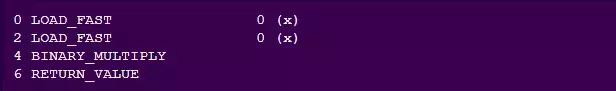该程序将 x 载入两次，执行 BINARY_MULTIPLY 操作，并且返回得到的值。math.pow()使用 dis.dis(lambda x: math.pow(x, 2)), 我们可以看到以下代码被执行: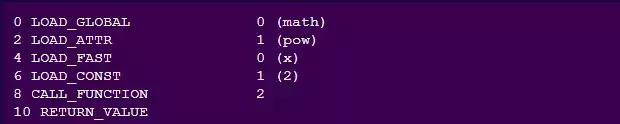math 模块从全局空间开始加载变量，然后加载pow属性。接下来，加载两个参数并调用pow函数，该函数会返回计算值。求幂使用dis.dis(lambda x: x ** 2), 我们可以看到以下代码被执行：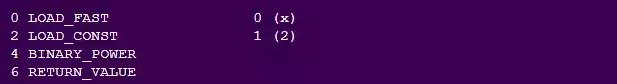该程序先加载 x, 再加载2，然后运行 BINARY_POWER 并返回计算结果。.BINARY_MULTIPLY 与 BINARY_POWER使用math.pow()函数作为一个比较点，乘法和求幂的字节码的只有一部分有所不同: 调用BINARY_MULTIPLY 与调用BINARY_POWER。BINARY_MULTIPLY这个函数位于这里(https://github.com/Python/cPython/blob/b509d52083e156f97d6bd36f2f894a052e960f03/Objects/longobject.c#L3645-L3665 )的Python源代码中。它做了一些有趣的事情: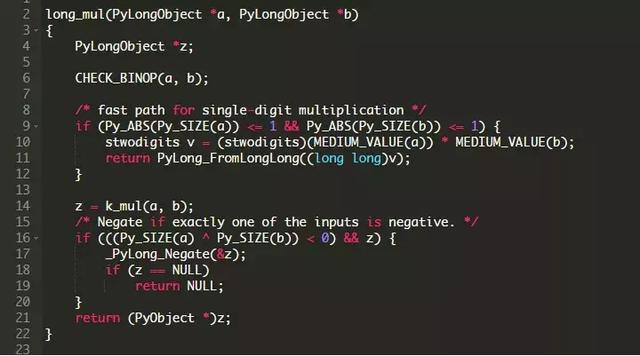对于较小的数，这个函数使用二进制乘法。对于较大的值，该函数使用Karatsuba乘法，这是一种针对较大数字的快速乘法算法。我们可以看到这个函数是如何在 ceval.c中被调用的: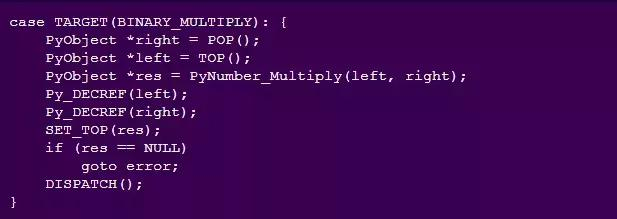BINARY_POWER这个函数位于这里(https://github.com/Python/cPython/blob/b509d52083e156f97d6bd36f2f894a052e960f03/Objects/longobject.c#L4118-L4305 )的Python源代码中。它还做了一些有趣的事情:源代码太长，所以无法完全包括进来，这在一定程度上解释了这种不利的性能。以下是一些有趣的代码片段: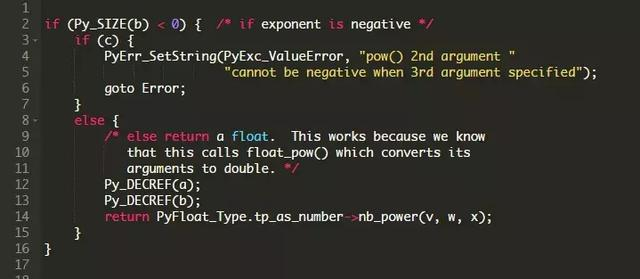在创建了一些指针之后，该函数会检查power给出的数是浮点数还是负数，在这里它可能会出错，也可能会调用另一个函数来处理求幂操作。如果两种情况都不是，该函数将检查第三个参数，根据ceval.c的代码来看这个参数通常是None：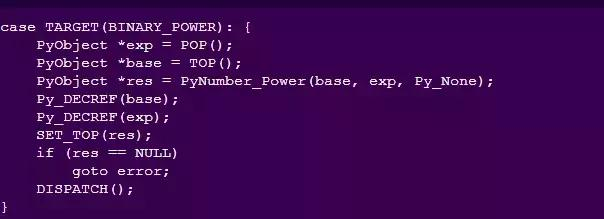最后，该函数定义了两个例程: REDUCE用于模降，MULT用于乘法和减法。乘法函数对两个值使用了long_mul函数，这与BINARY_MULTIPLY中使用的函数相同。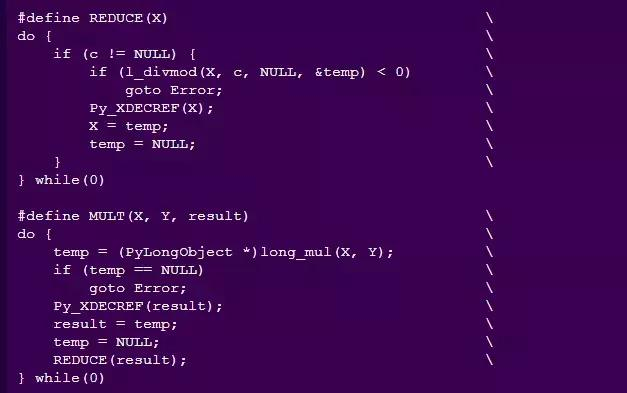之后，该函数使用《应用密码学手册》第14.6章中定义的从左到右k次求幂方法: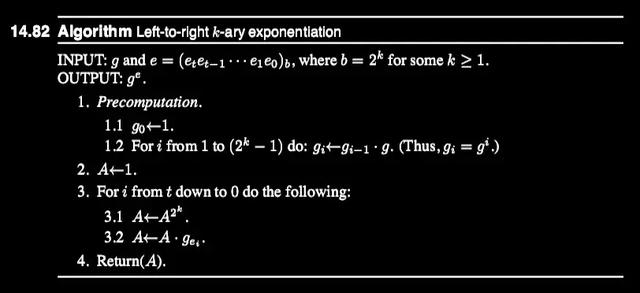图表化性能差异我们可以使用上面的timeit库分析在不同值时的代码，并查看性能如何随时间变化。生成函数为了测试不同power值下的性能，我们需要生成一些函数。math.pow()和取幂由于这两个函数都已经在Python源代码中，所以我们需要做的就是定义一个求幂函数，并且我们可以在一个timeit调用中来调用它。“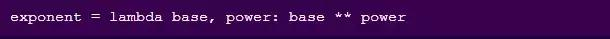连乘由于每一次power发生变化时，这个函数也会发生变化。所以每一次函数中的 base 发生变化时，我们都需要生成一个新的乘法函数。为此，我们可以生成一个像 x*x*x 这样的字符串，并对它调用eval()来返回一个函数: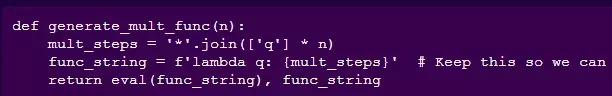这样，我们就可以像这样来创建一个 multiply 函数：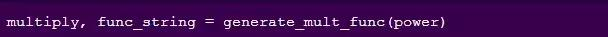如果我们调用 generate_mult_func(4), multiply函数将会是一个类似于这样的匿名函数：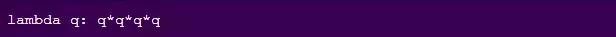查找交叉点使用这里(https://repl.it/@reagentx/Find-Crossover )贴出的代码，我们可以决定 multiply 在什么情况下会变得比 exponent 效率更低。我们先从这些值开始：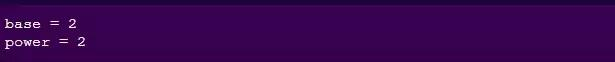我们持续循环，直到该函数执行100,000次 multiply 迭代的时间比执行100,000次 exponent 迭代的时间慢。首先，这里是计时时间，使用math.pow()作为一个比较点:当我们在repl.it上运行时, Python在1.2s内找到了交叉点: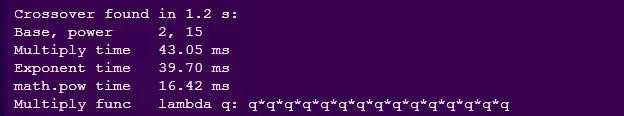因此，在我们的表达式达到2^14时，连乘是运行最快的，而在到达2^15时, 求幂变成最快的。图表化性能。使用Pandas, 我们可以跟踪每一次求幂的时间：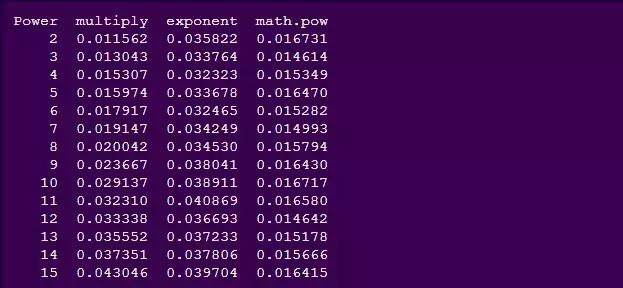使用下面的代码来生成一条折线图是非常简单的：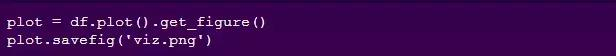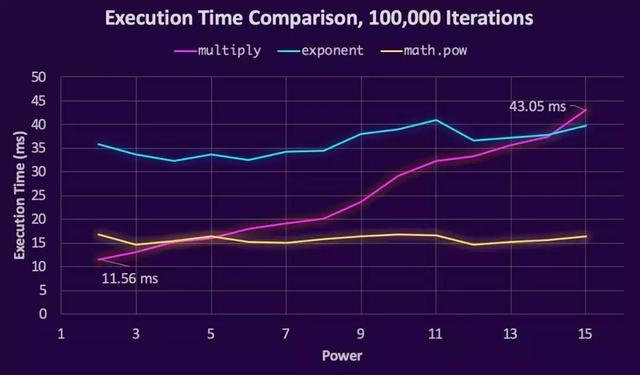有趣的是, math.pow() 和 exponent 的大多数操作都是以相同的速率来执行的 ,而我们的multiply函数差异很大。不出所料，乘法链越长，执行所需的时间就越长。更多的性能测试虽然交叉很有趣，但这并没有显示幂大于15时的情况。幂上升到1000，我们得到以下趋势: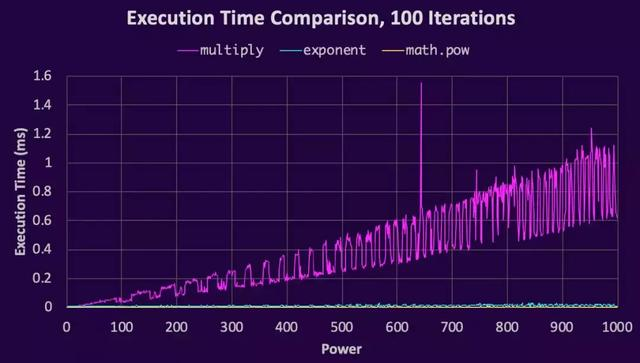当我们放大以使math.pow()和exponent更明显时，我们看到它们有相同的性能趋势并仍在继续: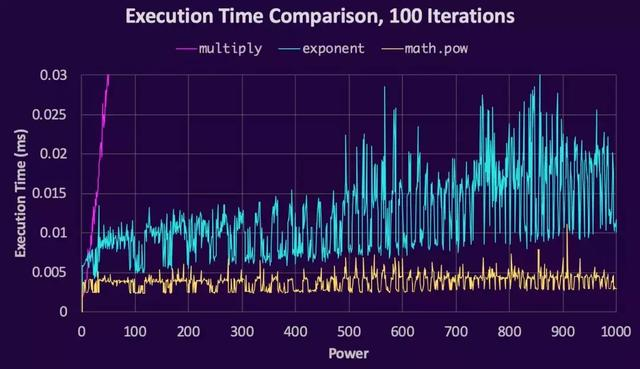虽然使用 ** 时时间会逐渐增加，但是math.pow()通常是以相同的速度执行。结论当编写使用小指数的算法时，这里证明为幂小于15时，进行连乘比使用**指数运算符更快。此外，在幂大于5的情况下，math.pow()比连乘更有效，而且它总是比**操作符更有效，所以没有任何理由去使用**。此外，在JavaScript中也是如此。感谢@juliancoleman在这里(https://jsperf.com/mult-vs-expo 0做的这个比较!英文原文：https://chrissardegna.com/blog/posts/python-expontentiation-performance/ 译者：野生大熊猫
展开全文• 既然文章是写给财务人员的，那么我就首先讲Python的数字。Python的数字有两类：整数，英文叫做：integer，如：1，3，6，20，100等等浮点数，英文叫做：float，如：1.2，6.73，1024.83等等基于数字的运算符：符号用途...
• pythonpow函数 Python pow()函数 (Python pow() function) pow() function is a library function in Python, is used to get the x to the power of y, where x is the base and y is the power – in other ...机器学习 深度学习 人工智能 java
• 幂运算是高更数学的应用学科，是一种关于幂的数学运算。...在python中就有内置函数pow函数表示幂的运算。1、pow()函数Python的内置函数，它计算并返回x的y次方的值。2、语法pow(x, y, z)3、参数x -- 数值表达式...
• 幂运算是高更数学的应用学科，是一种关于幂的数学运算。...在python中就有内置函数pow函数表示幂的运算。1、pow()函数Python的内置函数，它计算并返回x的y次方的值。2、语法pow(x, y, z)3、参数x -- 数值表达式...
• 一、最朴素的方法和pow比较python中求两个a的b次方，常见的方法有:pow(a,b),a**b。那么这两个是否有区别，而且他们底层是怎么实现的呢？最容易想到的方法就是：循环b次，每次都乘以a。但是究竟底层是不是这样实现的...
• 以下是 math 模块 pow() 方法的语法:importmathmath.pow(x,y)内置的 pow() 方法pow(x,y[,z])函数是计算x的y次方，如果z在存在，则再对结果进行取模，其结果等效于pow(x,y) %z注意：pow() 通过内置的方法直接调用，...
• 幂运算是高更数学的应用学科，是一种关于幂的数学运算。...在python中就有内置函数pow函数表示幂的运算。1、pow()函数Python的内置函数，它计算并返回x的y次方的值。2、语法pow(x, y, z)3、参数x -- 数值表达式...
• 这篇文章我们来讲一下在网站建设中，pythonpow函数用法及功能说明。本文对大家进行网站开发设计工作或者学习都有一定帮助，下面让我们进入正文。幂运算是高更数学的应用学科，是一种关于幂的数学运算。同底数幂...
• 幂运算是高更数学的应用学科，是一种关于幂的数学运算。...在python中就有内置函数pow函数表示幂的运算。1、pow()函数Python的内置函数，它计算并返回x的y次方的值。2、语法pow(x, y, z)3、参数x -- 数值表达式...
• 幂运算是高更数学的应用学科，是一种关于幂的数学运算。...在python中就有内置函数pow函数表示幂的运算。1、pow()函数Python的内置函数，它计算并返回x的y次方的值。2、语法pow(x, y, z)3、参数x -- 数值表达式...
• 英文文档(x,y[,z])Returnxto the powery; ifzis present, returnxto the powery,moduloz(computed more efficiently... The two-argumentform is equivalent to using the power operator: .pow()pow(x, y[, z])返回...
• 本文实例讲述了Pythonpow()和math.pow()函数用法。共享给大家供大家参考，详细如下：1. 内置函数pow()>>> help(pow)Help on built-in function pow in module __builtin__:pow(...)pow(x, y[, z]) -> ...
• 摘要:下文讲述Python代码中pow()函数的功能说明，如下所示:pow函数功能说明pow() 函数用于计算 xy(x 的 y 次方) 的值pow函数语法:import mathmath.pow( x, y ):用于计算x的y次方或pow(x, y[, z]):用于计算x的y次方，...
• 语法以下是 math 模块 pow() 方法的语法:import mathmath.pow( x, y )内置的 pow() 方法pow(x, y[, z])函数是计算x的y次方，如果z在存在，则再对结果进行取模，其结果等效于pow(x,y) %z注意：pow() 通过内置的方法...
• 幂运算是高更数学的应用学科，是一种关于幂的数学运算。...在python中就有内置函数pow函数表示幂的运算。1、pow()函数Python的内置函数，它计算并返回x的y次方的值。2、语法pow(x, y, z)3、参数x -- 数值表达式...
• 本文实例讲述了Pythonpow()和math.pow()函数用法。分享给大家供大家参考，具体如下：1. 内置函数pow()>>> help(pow)Help on built-in function pow in module __builtin__:pow(...)pow(x, y[, z]) -> ...
• 幂运算是高更数学的应用学科，是一种关于幂的数学运算。...在python中就有内置函数pow函数表示幂的运算。1、pow()函数python的内置函数，它计算并返回x的y次方的值。2、语法pow(x, y, z)3、参数x -- 数值表达式...
• python powPython pow() function usually takes two number arguments and returns their power. Python pow（）函数通常采用两个数字参数并返回其幂。...Python pow() function syntax is: Python pow（）函数...机器学习 人工智能 c++ java
• 幂运算是高更数学的应用学科，是一种关于幂的数学运算。...在python中就有内置函数pow函数表示幂的运算。1、pow()函数Python的内置函数，它计算并返回x的y次方的值。2、语法pow(x, y, z)3、参数x -- 数值表达式...
• 幂运算是高更数学的应用学科，是一种关于幂的数学运算。...在python中就有内置函数pow函数表示幂的运算。1、pow()函数Python的内置函数，它计算并返回x的y次方的值。2、语法pow(x, y, z)3、参数x -- 数值表达式...
• 一.pow函数介绍在python中内置函数pow()一共有两个参数，x和y，并返回 xy（x的y次方） 的值，语法如下：pow(x, y)参数介绍：x — 数值表达式(整数或者浮点数)； y — 数值表达式(整数或者浮点数)； z — 数值表达式......

# pow函数pythonpython 订阅